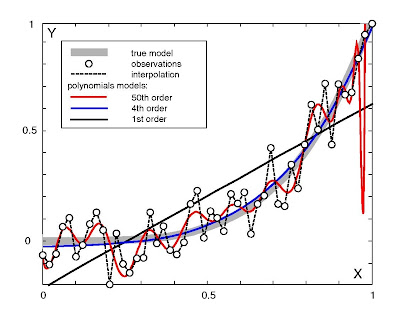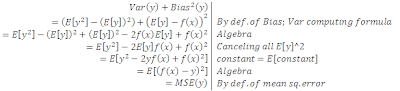# Conceptualizing Learning ErrorMarch 1, 2009
720 Views

When you are trying to find the correct equation to model and predict financial data, you will always have some error. If you are using regression to predict the next period’s return, you will probably measure the accuracy by mean squared error (MSE).

Error can be broken down into two components, and these two components can be interpreted as the sources of the error. Error = Bias + Variance

1) Bias is the error incurred by the expected prediction relative to the optimal/true prediction (Bias = E[y]-f(x), where f(x) is the true prediction and y is the approximation).
For example, using a 1st degree polynomial (a line) to approximate a 2nd degree polynomial (a parabola) will intrinsically have some bias error because a line cannot match a polynomial at all points.

2) Variance is the average error compared to expected prediction (Var = E[(y-E[y])^2]).
For example, if you only have 2 two sample data points, the function class of all 1st degree polynomials (ax+b) containing those two points will have no variance because only one line can go through the two points. However, the function class of all 2nd degree polynomials (ax2+bx+c) will have higher variance because there are infinite parab

When you are trying to find the correct equation to model and predict financial data, you will always have some error. If you are using regression to predict the next period’s return, you will probably measure the accuracy by mean squared error (MSE).

Error can be broken down into two components, and these two components can be interpreted as the sources of the error. Error = Bias + Variance

1) Bias is the error incurred by the expected prediction relative to the optimal/true prediction (Bias = E[y]-f(x), where f(x) is the true prediction and y is the approximation).
For example, using a 1st degree polynomial (a line) to approximate a 2nd degree polynomial (a parabola) will intrinsically have some bias error because a line cannot match a polynomial at all points.

2) Variance is the average error compared to expected prediction (Var = E[(y-E[y])^2]).
For example, if you only have 2 two sample data points, the function class of all 1st degree polynomials (ax+b) containing those two points will have no variance because only one line can go through the two points. However, the function class of all 2nd degree polynomials (ax2+bx+c) will have higher variance because there are infinite parabolas that can be strung through two points. Therefore you will have higher generalization error when you test on out-of-sample data. Here’s a picture of both examples, focus on the 1st order and 50th order, clearly both will have high prediction error:Now that I’ve covered the intuition, here’s the derivation of Bias and Variance from MSE, working backwards, with justifications for each step (click to enlarge):The bias-variance tradeoff is a fundamental, intrinsic challenge for machine learning. If you are using a neural network, you will have to deal with very high variance; more nodes = more variance + less bias. If you are using linear regression, you will have to accept very high bias.

I have glossed over noisy data, which makes the decomposition MSE = Bias + Var + Noise. However, I think it’s more interesting to imagine that noise doesn’t exist, and actually we just don’t have a good enough model yet. For example, you could call a coin flip random, but I think it’s deterministic based on launch velocity, air resistance, wind, etc and we just don’t have the capability to measure and predict these complicating factors. That’s a philosophical question. Of course treating un-model-able factors as noise is a very useful simplifying assumption. Google “bias variance” for further info and more on adding in a noise factor if you’re interested.

I think next I will do a short series on the three sources of overfitting/data snooping procedural flaws: training on the test data, survivorship bias, and overfitting the out-of sample test set. The last is the most challenging to watch out for and the least well-known.

Please leave comments or corrections on bias/variance or anything else.